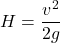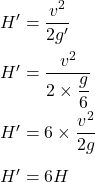## . How many times higher could an astronaut jump on the Moon than on Earth if her takeoff speed is the same in both locations (gravitational

Question

. How many times higher could an astronaut jump on the Moon than on Earth if her takeoff speed is the same in both locations (gravitational acceleration on the Moon is about on-sixth of that on Earth)?
1) On the Moon, the jump height is about 3 times higher than on Earth.
2) On the Moon, the jump height is about 6 times higher than on Earth.
3) On the Moon, the jump height is about 36 times higher than on Earth.
4) On the Moon, the jump height is more than on Earth by about a times.

in progress 0
2 weeks 2021-09-02T08:58:58+00:00 1 Answers 0 views 0

On the Moon, the jump height is about 6 times higher than on Earth.

Explanation:

The maximum height reached by an object is given by the formula as follows :v is the speed of object

g is acceleration due to gravity on Earth’s surface

If g’ is acceleration on surface of Moon, g’ = g/6

Let H’ is the height reached by object on Moon, then,On the Moon, the jump height is about 6 times higher than on Earth. Hence, the correct option is (2).